# Negative Poisson's ratio materials

### Meaning of Poisson's ratioNegative stiffness inclusions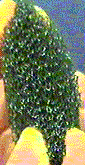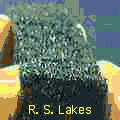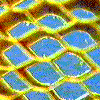Preamble
Poisson's ratio, also called the Poisson coefficient, is the ratio of transverse contraction strain to longitudinal extension strain in a stretched bar. Since most common materials become thinner in cross section when stretched, Poisson's ratio for them is positive. The reason is that inter-atomic bonds realign with deformation. Stretching of normal honeycomb, shown on the right, illustrates the concept. Normal polymer foams or cellular solids, above left, have a positive Poisson's ratio. Re-entrant polymer foams developed in our laboratory, above right, have a negative Poisson's ratio. After our original article appeared in Science, they were called anti-rubber by James Glieck of the New York Times, and were called auxetic (or auxetics, or auxetic materials) by K. Evans and co-workers in Exeter, England, and F. Scarpa and co-workers in Bristol, England, and were called dilational by mathematician Graeme Milton of the University of Utah. We have never called them metamaterials, though such terminology has recently become popular in referring to materials that attain interesting or extreme properties via structure rather than composition. Similarly we did not use the phrase materials by design. Negative Poisson's ratio chiral honeycomb, above center, unrolls when stretched; it is isotropic in two dimensions. Click on the image for the article. Negative Poisson's ratio re-entrant (bow-tie) honeycomb, below right, unfolds when stretched; it is isotropic in two dimensions only for certain lengths and angles. Bow-tie honeycomb was introduced by Kolpakov and by Schajer, Robertson, et al.; see work by others link above.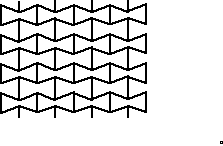The original article. " Foam structures with a negative Poisson's ratio", Science, 235 1038-1040 (1987).
This is the first designed negative Poisson's ratio material and is the first one that can be isotropic. Bottom: recent articles

Summary
A novel foam structure is presented, which exhibits a negative Poisson's ratio. Such a material expands laterally (gets fatter) when stretched. See the upper foam (cellular solid) in the above image. Such behavior is in contrast to ordinary materials such as the lower foam in the image above. For example, rubber has a Poisson's ratio approaching the isotropic upper limit 0.5 and therefore becomes substantially thinner when stretched. Negative Poisson ratio solids easily undergo volume changes. By contrast, rubbery materials easily undergo shape changes (shear deformation) but are much stiffer in relation to volume changes. The distinction is shown in a map adapted from Milton. The idealized unit cell from the original Science article appeared on the front page of the New York Times as shown on the right; see also other publicity.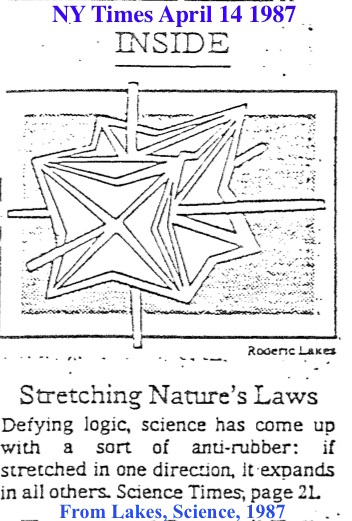Foams with negative Poisson's ratios were produced from conventional low density open-cell polymer foams by causing the ribs of each cell to permanently protrude inward, resulting in a re-entrant structure. Specimens of conventional foam were compressed triaxially, i.e. in three orthogonal directions, and were placed in a mold. The mold was heated to a temperature slightly above the softening temperature of the foam material. Stress strain curves and curves of nonlinear behavior are given below. These foams are essentially isotropic; they do not depend on anisotropy to achieve the effects observed. Applications of novel, re-entrant foams with negative Poisson's ratios may be envisaged in view of the above. An example of the practical application of a particular value of Poisson's ratio is the cork of a wine bottle. The cork must be easily inserted and removed, yet it also must withstand the pressure from within the bottle. Rubber, with a Poisson's ratio of 0.5, could not be used for this purpose because it would expand when compressed into the neck of the bottle and would jam. Cork, by contrast, with a Poisson's ratio of nearly zero, is ideal in this application. It is anticipated that re-entrant foams may be used in such applications as sponges, robust shock absorbing material, air filters, biomaterials and fasteners. Negative Poisson's ratio effects can result from non-affine deformation, from certain chiral microstructures, on an atomic scale, or from structural hierarchy. Negative Poisson's ratio materials can exhibit slow decay of stress according to Saint-Venant's principle. Later writers have called such materials anti-rubber, auxetic (auxetics), or dilatational. These materials are an example of extremal materials. They have also been called metamaterials by later writers. Some recent writers use the word architected materials or architectured materials. Combined foam animation shows normal and re-entrant foams together. Bottom: recent articles.

Patents.
There are several U. S. patents as well as Canada and overseas patents.
If you are interested in commercial applications, please contact the Wisconsin Alumni Research Foundation (WARF), for information on U. S. and international patents and licensing.

Directions for making foam: How to do it.

Top

## Journal articles and abstracts

Lakes, R. S.,"Foam structures with a negative Poisson's ratio", Science, 235 1038-1040 (1987). See summary above.

Lakes, R. S., "Negative Poisson's ratio materials", Science, 238 551 (1987).
The negative Poisson's ratio effect is not due to Cosserat elasticity. The classical theory of elasticity has no length scale. Negative Poisson's ratio is classically attainable and does not require the characteristic length scale present in Cosserat or micropolar elasticity. Get pdf or gif. Further details are given here on Poisson's ratio in linear isotropic elasticity.

Friis, E. A., Lakes, R. S., and Park, J. B., "Negative Poisson's ratio polymeric and metallic foams", Journal of Materials Science, 23, 4406-4414 (1988).
Foam materials based on metal and several polymers were transformed so that their cellular architectures became re-entrant, ie. with inwardly protruding cell ribs. Foams with re-entrant structures exhibited negative Poisson's ratios as well as greater resilience than conventional foams. Foams with negative Poisson's ratios were prepared using different techniques and materials and their mechanical behavior and structure evaluated. Get preprint; reprint.

Chen, C. P. and Lakes, R. S., "Dynamic wave dispersion and loss properties of conventional and negative Poisson's ratio polymeric cellular materials", Cellular Polymers, 8(5), 343-359 (1989).
This article describes experimental investigations of the dynamical behaviour of conventional and negative Poisson's ratio foamed materials in torsional vibration. Dispersion of standing waves and cut-off frequencies were observed. Consequently, foamed materials do not obey the classical theory of elasticity or viscoelasticity. The dynamical effects were attributed to micro-vibrations of the cell ribs in a structural view and were associated with microstructure or micromorphic elasticity in a continuum view. Cut-off frequencies were lower in re-entrant foams with negative Poisson's ratios than in the conventional foams from which they were derived. An analytical structural model was developed in which the ribs of the conventional foams were modeled as free-free vibrating beams. The predicted cut-off frequencies were comparable to those observed experimentally.
In more recent parlance, these materials may be referred to as locally resonant metamaterials. Get pdf

In 1989 the term auxetic was introduced by a British group in connection with a proposal for a two dimensional molecular structure with a negative Poisson's ratio based on macroscopic bow tie structure of negative Poisson's ratio honeycomb.

Chen, C. P. and Lakes, R. S., "Holographic study of conventional and negative Poisson's ratio metallic foams: elasticity, yield, and micro-deformation", J. Materials Science, 26, 5397-5402 (1991)
This article presents an experimental study by holographic interferometry of the following material properties of conventional and negative Poisson's ratio copper foams: Young's moduli, Poisson's ratios, yield strengths, and characteristic lengths associated with inhomogeneous deformation. The Young's modulus and yield strength of the conventional copper foam were comparable to those predicted by microstructural modelling on the basis of cellular rib bending. The re-entrant copper foam exhibited a negative Poisson's ratio as indicated by the elliptic contour fringes on the specimen surface in the bending tests. Inhomogeneous, non-affine deformation was observed holographically in both foam materials. Get pdf; holographic bend fringe images.

Choi, J. B. and Lakes, R. S., "Design of a fastener based on negative Poisson's ratio foam", Cellular Polymers, 10, 205-212 (1991).
In this article we make use of the negative Poisson's ratio of recently developed cellular solids or spongy materials in the design of a press-fit fastener. Insertion of the fastener is facilitated by the lateral contraction which negative Poisson's ratio materials exhibit under compression. Removal of the fastener is resisted by the corresponding elastic expansion under tension. Get pdf

Lakes, R. S., "Experimental micro mechanics methods for conventional and negative Poisson's ratio cellular solids as Cosserat continua", J. Engineering Materials and Technology, 113, 148-155 (1991).
Continuum representations of micromechanical phenomena in structured materials are described, with emphasis on cellular solids. These phenomena are interpreted in light of Cosserat elasticity, a generalized continuum theory which admits degrees of freedom not present in classical elasticity. These are the rotation of points in the material, and a couple per unit area or couple stress. Experimental work in this area is reviewed, and other interpretation schemes are discussed. The applicability of Cosserat or micropolar elasticity to cellular solids and fibrous composite materials is considered as is the application of related generalized continuum theories. New experimental results are presented for foam materials with negative Poisson's ratios. Get pdf

Top

Lakes, R. S., "Deformation mechanisms of negative Poisson's ratio materials: structural aspects", J. Materials Science, 26, 2287-2292 (1991).
Poisson's ratio in materials is governed by the following aspects of the microstructure: the presence of rotational degrees of freedom, non-affine deformation kinematics, or anisotropic structure. Several structural models are examined. The non-affine kinematics are seen to be essential for the production of negative Poisson's ratios for isotropic materials containing central force linkages of positive stiffness. Non-central forces combined with pre-load can also give rise to a negative Poisson's ratio in isotropic materials. A chiral microstructure with non-central force interaction or non-affine deformation can also exhibit a negative Poisson's ratio. Toughness and damage resistance in these materials may be affected by the Poisson's ratio itself, as well as by generalized continuum aspects associated with the microstructure.

This article provides the conceptual framework for a wide variety of auxetic materials.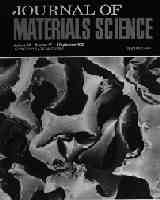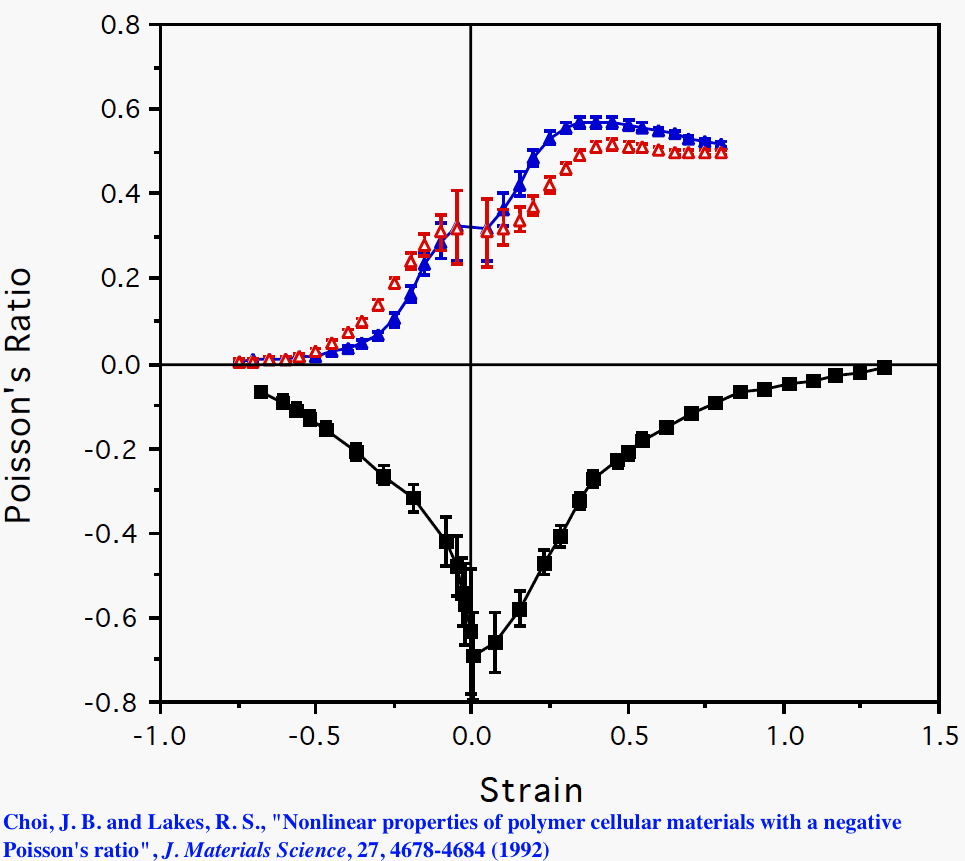Choi, J. B. and Lakes, R. S., "Nonlinear properties of polymer cellular materials with a negative Poisson's ratio", J. Materials Science, 27, 4678-4684 (1992). Cover issue.
Negative Poisson's ratio polymeric cellular solids (re-entrant foams) were studied to ascertain the optimal processing procedures which give rise to the smallest value of Poisson's ratio. The nonlinear stress - strain relationship was determined for both conventional and re-entrant foams; it depended upon the permanent volumetric compression achieved during the processing procedure. Poisson's ratio measured as a function of strain was found to have a relative minimum at small strains. The toughness of re-entrant foam increased with permanent volumetric compression, hence density. Stress-strain curve Diagram. Get pdf

Lakes, R. S., "The time dependent Poisson's ratio of viscoelastic cellular materials can increase or decrease", Cellular Polymers, 11, 466-469, (1992).
In viscoelastic materials, the Poisson's ratio is not a material constant but can depend upon time. For polymeric solids, the shear modulus relaxes much more than the bulk modulus, therefore, the Poisson's ratio nu (t) is an increasing function of time. In this article we demonstrate that such time dependence is not a necessary consequence of the theory of viscoelasticity. Viscoelastic composite microstructures are presented which result in nu(t) which decreases with time. Get pdf

Lakes, R. S., "Saint Venant end effects for materials with negative Poisson's ratios", J. Applied Mechanics, 59, 744-746 (1992). Get pdf.
In this article we analyze Saint-Venant end effects for materials with negative Poisson's ratios. We present an example of slow decay of stress arising from self-equilibrated stress at the end of a circular cylinder of elastic material with a negative Poisson's ratio. By contrast a sandwich panel containing rigid face sheets and a compliant core exhibits no anomalous effects for negative Poisson's ratio, but exhibits slow stress decay for core Poisson's ratio approaching 0.5. In sandwich panels with stiff but not perfectly rigid face sheets, slow decay of stress is known to occur; a negative Poisson's ratio results in end stress decay which is faster than it would be otherwise.

Choi, J. B. and Lakes, R. S., "Nonlinear properties of metallic cellular materials with a negative Poisson's ratio", J. Materials Science, 27, 5373-5381 (1992).
Negative Poisson's ratio copper foam was prepared and characterized experimentally. The transformation into re-entrant foam was accomplished by applying sequential permanent compressions above the yield point to achieve a triaxial compression. The Poisson's ratio of the re-entrant foam depended on strain and attained a relative minimum at strains near zero. Poisson's ratio as small as -0.8 was achieved. The strain dependence of properties occurred over a narrower range of strain than in the polymer foams studied earlier. Annealing of the foam resulted in a slightly greater magnitude of negative Poisson's ratio and greater toughness at the expense of a decrease in the Young's modulus. Get pdf; metal auxetics.

Lakes, R. S., "No contractile obligations", Nature, 358, 713-714, (1992).
Stretch most materials and you will expect to see their cross section shrink. But Alderson and Evans have synthesized a microporous polymer that swells as it is stretched, and Milton has designed a laminated composite that does the same. These advances in the newly developing science of materials with a negative Poisson's ratio promise improved control of properties and open the door to a new class of applications. Get pdf

Lakes, R. S., "Design considerations for negative Poisson's ratio materials" ASME Journal of Mechanical Design, 115, 696-700, (1993).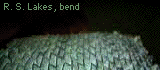This article presents a study of the implications of negative Poisson's ratios in the design of load bearing structural elements. Stress concentration factors are reduced in some situations, and unchanged or increased in others, when the Poisson's ratio becomes negative. Stress decay according to Saint Venant's principle can occur more or less rapidly as the Poisson's ratio decreases. Several design examples are presented, including a core for a curved sandwich panel and a flexible impact buffer.
Get pdf
Curvature of negative Poisson's ratio honeycomb during bending is convex in contrast to the saddle shape usually seen for positive Poisson's ratio, and is shown in the animation at the right and in this video

Top

Lakes, R. S., "Advances in negative Poisson's ratio materials" , Advanced Materials (Weinheim, Germany), 5, 293-296, (1993).
This article presents a review of recent developments in negative Poisson's ratio materials based on foams, laminates, microporous materials, and materials with structural hierarchy. Get pdf. By this time the term auxetic material had been introduced.

Rosakis, P., Ruina, A., and Lakes, R. S., "Microbuckling instability in elastomeric cellular solids", J. Materials Science, 28, 4667-4672 (1993).
Compressive properties of elastic cellular solids are studied via experiments upon foam and upon single cell models. Open cell foam exhibits a monotonic stress-strain relation with a plateau region; deformation is localized in transverse bands. Single cell models exhibit a force-deformation relation which is not monotonic. In view of recent concepts of the continuum theory of elasticity, the banding instability of the foam in compression is considered to be a consequence of the non-monotonic relation between force and deformation of the single cell. Get pdf

The negative stiffness represents an instability that can be viewed as a phase transition. Such instability occurs in foams as well as in fiber networks.

Chen, C. P. and Lakes, R. S., "Viscoelastic behaviour of composite materials with conventional or negative Poisson's ratio foam as one phase", J. Materials Science, 28, 4288-4298, (1993).
This article describes experimental investigations of viscoelastic properties of composites consisting of conventional and re-entrant negative Poisson's ratio copper foam as a matrix, with high loss filler materials: viscoelastic elastomer, solder, and indium. Viscoelastic properties of gallium and several ferrites were determined as well. The loss tangent of the copper- elastomer composite substantially exceeded the (lower) Voigt limit; the loss tangent of the copper-solder and copper-indium composites were close to the (upper) Hashin limit for two solid phases and one pore phase.

Chen, C. P. and Lakes, R. S., "Holographic study of non-affine deformation in copper foam with a negative Poisson's ratio -0.8", Scripta Metall et Mater., 29, 395-399, (1993).
Micro-deformation studies of conventional and negative Poisson's ratio copper foams were conducted holographically. Inhomogeneous, non-affine deformation was observed holographically in both foam materials. The negative Poisson's ratio material with a permanent volumetric compression ratio 2.2 exhibited a substantially greater non-affine deformation than the conventional material, in contrast to foam with compression ratio 3.0 examined earlier. Get pdf

Lakes, R. S. and Elms, K., "Indentability of conventional and negative Poisson's ratio foams", J. Composite Materials, 27,1193-1202, (1993).
The indentation resistance of foams, both of conventional structure and of a novel re-entrant structure giving rise to negative Poisson's ratio, was studied using holographic interferometry. In holographic indentation tests, re-entrant foams had higher yield strengths sigma y and lower stiffness E than conventional foams of the same original relative density. Damage in both kinds of foam occurred primarily directly under the indenter. Calculated energy absorption for dynamic impact is considerably higher for re-entrant foam than conventional foam.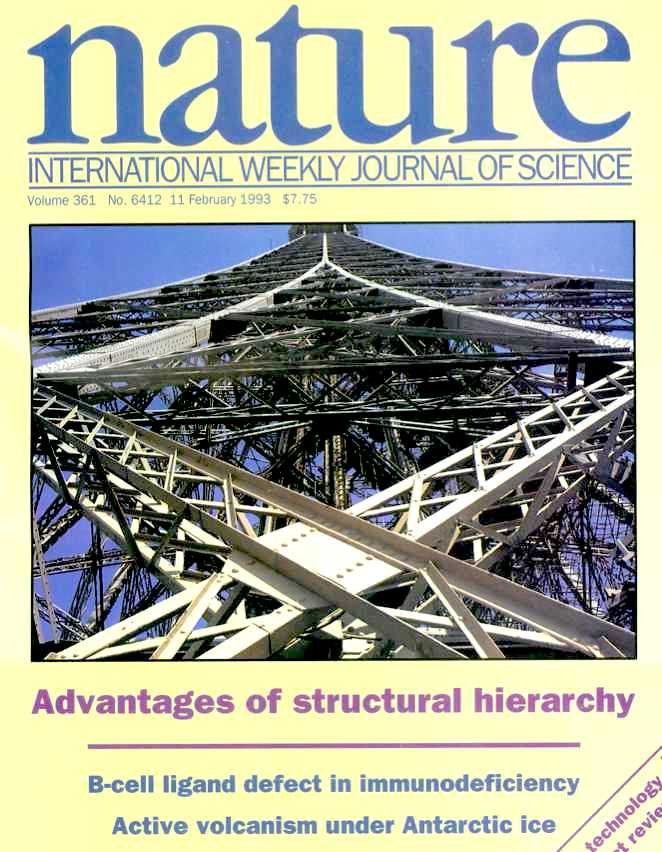Lakes, R. S., "Materials with structural hierarchy", Nature, 361, 511-515 (1993). Cover issue.
New hierarchical microstructures are presented for cellular materials, in which the structural elements themselves are cellular. The compressional strength of low density hierarchical materials can be thousands of times greater than that of a conventional cellular material of the same density. The use of hierarchical structure to achieve negative Poisson's ratio is reviewed.

Choi, J. B. and Lakes, R. S., "Nonlinear analysis of the Poisson's ratio of negative Poisson's ratio foams", J. Composite Materials, 29, (1),113-128, (1995).
This article contains analytic study of Poisson's ratio of re-entrant foam materials with negative Poisson's ratio. These materials get fatter when stretched and thinner when compressed. The Poisson effect is so fundamentally important to the properties of a material that a large change in the value of the ratio will have significant effects on the material's mechanical performance. Isotropic foam structures with negative Poisson's ratio have been fabricated through a permanent volumetric transformation. The cells were converted from the convex polyhedral shape of conventional foam cells to a concave or "re-entrant" shape. Mechanical behavior of a re-entrant open cell foam material will differ from that of a conventional foam in ways not addressed by existing theoretical treatment. Poisson's ratio as a function of strain is obtained by modeling the three dimensional unit cell as an idealized polyhedron unit cell. Poisson's ratio is predicted to approach the isotropic limit of -1 with increasing permanent volumetric compression ratio of idealized cells, in comparison with experimental values as small as - 0.8. Get pdf

Top

Chen, C. P., and Lakes, R. S., "Micromechanical analysis of dynamic behavior of conventional and negative Poisson's ratio foams", J. Engineering Materials and Technology, 118, 285-288 (1996).
Both conventional and negative Poisson's ratio foams exhibit dispersion of acoustic waves as well as cut-off frequencies at which the group velocity tends to zero. This macroscopic behavior is attributed to micro-vibration of the cell ribs. The purpose of this article is to develop a micromechanical model of the cut-off frequency. This model is based on the resonance of ribs which may be straight, curved, or convoluted. Get pdf

Martz, E. O., Lee, T., Lakes, R. S., Goel, V. K. and Park, J. B. "Re-entrant transformation methods in closed cell foams", Cellular Polymers, 15, 229-249, (1996).
Open-cell foams have been transformed in prior studies, to a re-entrant (inward bulging) cell structure which gives rise to a negative Poisson's ratio. Different methods were used for polymer foams with and without a softening point. In this study, methods based on air pressure were developed for the transformation of closed cell polymer foams. L ow density polyethylene foam was successfully transformed, however polymethacrylimide foam was densified without transformation. Get pdf, image figures

Martz, E. O., Lakes, R. S., and Park, J. B. "Hysteresis behaviour and specific damping capacity of negative Poisson's ratio foams", Cellular Polymers, 15, 349-364, (1996). Open cell polyurethane foams have been previously made to be re-entrant, in that their cell ribs are inwardly bent, which results in the foam exhibiting a negative Poisson's ratio. Untransformed control and transformed negative Poisson's ratio foams were cyclically loaded at various levels of pre-strain to determine their hysteresis behavior, and from this, to elucidate its specific damping characteristics. Both untransformed and transformed foams exhibited non-linear hysteresis behavior at high cyclic strains, regardless of their initial levels of pre-strain. The specific damping capacity of the transformed foam with extreme compressive pre-strain was higher than that of the untransformed foam. This effect was attributed to the rubbing of the inwardly buckled cell ribs of the transformed foam. The specific damping capacity varied with both the level of pre-strain and with the amplitude of cyclic strain about that level. Get pdf

Choi, J. B. and Lakes, R. S., "Fracture toughness of re-entrant foam materials with a negative Poisson's ratio: experiment and analysis", Int. J. Fracture, 80, 73-83, (1996).
Fracture toughness of re-entrant foam materials with a negative Poisson's ratio is explored experimentally as a function of permanent volumetric compression ratio, a processing variable. Values of J toughness of negative Poisson's ratio open cell copper foams are enhanced by 80%, 130%, and 160% for permanent volumetric compression ratio of 2.0, 2.5, and 3.0, respectively, compared to the J value of the conventional foam (with a positive Poisson's ratio). Analytical studies are based on idealized polyhedral cell structures approximating the shape of the conventional and re-entrant cells. For conventional foam, analysis shows toughness increasing with density. For re-entrant foam, the analysis shows toughness increasing as Poisson's ratio becomes more negative.
Get pdf

Prall, D. and Lakes, R. S., "Properties of a chiral honeycomb with a Poisson's ratio of -1", Int. J. of Mechanical Sciences, 39, 305-314, (1997).A theoretical and experimental investigation is conducted of a two-dimensionally chiral honeycomb. The honeycomb exhibits a Poisson's ratio of -1 for deformations in-plane. This Poisson's ratio is maintained over a significant range of strain, in contrast to the variation with strain seen in known negative Poisson's ratio materials. This is the first two-dimensional metamaterial that is chiral; we did not call it by such a name. Chirality is also called hemitropy or noncentrosymmetry. Remark: these chiral honeycombs, also called chiral lattices, have been analyzed as Cosserat elastic solids.
A. Spadoni, M. Ruzzene, Elasto-static micropolar behavior of a chiral auxetic lattice, Journal of the Mechanics and Physics of Solids, 60, 156-171 (2012).
X. N. Liu, G. L. Huang, G. K. Hu, Chiral effect in plane isotropic micropolar elasticity and its application to chiral lattices, Journal of the Mechanics and Physics of Solids, 60, 1907-1921 (2012).

Lee, T. and Lakes, R. S., "Anisotropic polyurethane foam with Poisson's ratio greater than 1", Journal of Materials Science, 32, 2397-2401, (1997).
Anisotropic polymer foams have been prepared, which exhibit a Poisson's ratio exceeding 1, and ratios of longitudinal to transverse stiffness exceeding 50. The foams are as much as 20 times stiffer in the longitudinal direction than the foams from which they were derived. The transformation process involved applying to open-cell polyurethane foam an axial strain of 25% to 45%, at a temperature above the softening point, followed by cooling under axial strain. Get pdf, Fig. 7, Fig. 8.

Top

Loureiro, M. A. and Lakes, R. S., "Scale-up of transformation of negative Poisson's ratio foam: Slabs, Cellular Polymers, 16, 349-363, (1997).
This paper presents an experimental study performed on the scale-up of processing of negative Poisson's ratio foam. These materials become thinner under compression and fatter under tension, opposite to ordinary materials. The transformation process consists of triaxial compression combined with heat treatment. Transformed foam cells have a concave or "re-entrant" shape. Scale-up is achieved by use of a modified mold assembled around the foam slab and longer processing times. Foam with a distribution of cell sizes was transformed in small block and large slab forms. These blocks and slabs exhibited a negative Poisson's ratio. Get pdf

Lakes, R. S. and Lowe, A. "Negative Poisson's Ratio Foam as Seat Cushion Material", Cellular Polymers, 19, 157-167, (2000).
Negative Poisson's ratio foam was used in the development of seat cushions which exert reduced peak pressure upon the skin of seated persons. Foam processing techniques were scaled up. A longer processing time was required for cushion size samples in comparison with small samples. Pressure distributions on a seated subject were measured using a pressure-sensitive array. Seated pressure distribution became more favorable with decreasing sample density for both conventional and re-entrant foam blocks. Foam thickness played a small role in the seated pressure performance of foam cushions. Re-entrant foam at densities of between 2 and 4 lb/ft3 (0.032 to 0.064 g/cm3) performed better (lower maximum seating pressure) than conventional foam samples of comparable density. Get pdf

Lakes, R. S., "Lateral Deformations in Extreme Matter", perspective, Science, 288, 1976, June (2000). article link, pdf

Lakes, R. S., "A broader view of membranes", Nature, 414, 503-504, 29 Nov. (2001). - pdf. Interpretation of an advance in auxetic membranes.

Brandel, B. and Lakes, R. S., "Negative Poisson's ratio polyethylene foams", J. Materials Science, 36, 5885-5893, July (2001). Various polyethylene foams were subjected to thermo-mechanical processing with the aim of transforming them into re-entrant materials exhibiting negative Poisson's ratio. Following transformation, large cell foams (cell sizes of 1 and 2 mm) exhibited re-entrant cell structure and negative Poisson's ratio over a range of processing times and temperatures. Poisson's ratio vs. strain for these foams was similar to prior results for reticulated polyurethane foams. Following processing, microcellular polyethylene foam was densified but cells remained convex; it did not exhibit a substantial negative Poisson's ratio. This foam had a different transition temperature as determined via DSC than the large cell foams. pdf

Wang, Y. C., Lakes, R. S., and Butenhoff, A., "Influence of cell size on re-entrant transformation of negative Poisson's ratio reticulated polyurethane foams", Cellular Polymers, 20: 373-385, (2001). Several foams of different cell-size, including Scott Industrial polyurethane foam with large cells (20 pores per inch, ppi, or 1.2 mm per pore, black), medium cells (65 ppi, or 0.4 mm per pore, green), and near-microcellular (100 ppi, 0.25 mm per pore, white), were processed over various time and temperature regimes to ascertain the role of cell size in transformation to negative Poisson's ratio materials. These foams were transformed successfully, and exhibited negative Poisson's ratio behavior. Poisson's ratio was measured using a new laser based setup. For all as-received (unprocessed) foams with different cell sizes, Poisson's ratio decreased with compressive axial strain and increased with tensile strain up to a maximum. The maximum Poisson's ratio in tension decreased as cell size increases. The strain at which maximum Poisson's ratio occurs, increased with cell size. In negative Poisson's ratio foams, minimum Poisson's ratios of -0.8, -0.5, and -0.4 for 20 ppi, 65 ppi, and 100 ppi foams, respectively were observed. pdf

Lakes, R. S. and Witt, R., "Making and characterizing negative Poisson's ratio materials", International Journal of Mechanical Engineering Education, 30, 50-58, Jan. (2002). We present an introduction to the use of negative Poisson's ratio materials to illustrate various aspects of mechanics of materials. Poisson's ratio is defined as minus the ratio of transverse strain to longitudinal strain in simple tension. For most materials, Poisson's ratio is close to 1/3. Negative Poisson's ratios are counterintuitive but permissible according to the theory of elasticity. Such materials can be prepared for classroom demonstrations, or made by students. pdf

Wang, Y. C. and Lakes, R. S., "Analytical parametric analysis of the contact problem of human buttocks and negative Poisson's ratio foam cushions", International Journal of Solids and Structures, 39, 4825-4838 Sept. (2002). Analytical investigations on the contact problems between two homogeneous and isotropic soft bodies were performed to simulate the contact of human buttocks and seat cushions. The cushion materials' Poisson's ratio were allowed to be negative. The human buttocks were modeled as an ideal sphere with radius 15 cm, and assumed to have a low Young's modulus and a Poisson's ratio close to 0.5. These parameters were held constant during our analysis. Peak contact pressure was reduced by adjusting the contour curvature of cushions according to Hertz theory, as expected. Moreover, analysis by both the Hertz model and a finite thickness 3D elasticity model showed that using negative Poisson's ratio cushions could further reduce the pressure. Negative Poisson's ratio cushions may be beneficial in the prevention of pressure sores or ulcers in the sick and in reduction of pressure-induced discomfort in seated people. pdf

Wang, Y. C. and Lakes, R. S., "Composites with inclusions of negative bulk modulus: extreme damping and negative Poisson's ratio", J. Composite Materials, 39, 1645-1657, (2005). The effect of a negative bulk modulus phase in elastic composites is studied. Negative bulk modulus K < 0 is shown to be possible in selected unit cells. In isotropic solids, K < 0 can be attained for negative Poisson's ratio sufficiently small, below the stability limit (for stress control) Poisson's ratio = -1. Such materials, if used as inclusions, are predicted to be stable with respect to band formation, even if they are large. Composites with spherical inclusions of negative bulk moduli are shown to exhibit negative Poisson's ratio and anomalies in composite bulk modulus and Young's modulus (and in the corresponding mechanical damping) but not in the shear modulus. pdf

Martz, E. O., Lakes, R. S., Goel, V. K. and Park, J. B. "Design of an artificial intervertebral disc exhibiting a negative Poisson's ratio", Cellular Polymers, 24, 127-138, (2005). An artificial intervertebral disc exhibiting an anisotropic negative Poisson's ratio has been designed and characterized in the laboratory. This disc prosthesis incorporates negative Poisson's ratio to prevent bulge which might impinge on nerves, as well as the duplication of compressive axial stiffness of the natural lumbar intervertebral disc. The disc is also compliant in bending and torsion. No claim is made regarding possible clinical benefit is made based on this laboratory research. No tests upon living animals or humans were done in this project. get pdf

Lakes, R. S. and Wineman, A., "On Poisson's ratio in linearly viscoelastic solids", Journal of Elasticity, 85, 45-63 (2006). Poisson's ratio in viscoelastic solids is in general a time dependent (in the time domain) or a complex frequency dependent quantity (in the frequency domain). We show that the viscoelastic Poisson's ratio has a different time dependence depending on the test modality chosen; interrelations are developed between Poisson's ratios in creep and relaxation. The difference, for a moderate degree of viscoelasticity, is minor. Correspondence principles are derived for the Poisson's ratio (also called the Poisson coefficient or Poissonzahl) in transient and dynamic contexts. The viscoelastic Poisson's ratio need not increase with time, and it need not be monotonic with time. Physical examples are given of material microstructures which give rise to designed time dependent Poisson's ratios. Some of these microstructures give rise to negative Poisson's ratio over part of the time scale. get pdf

Shang, X. and Lakes, R. S., "Stability of elastic material with negative stiffness and negative Poisson's ratio", Physica Status Solidi (b), 244, 1008-1026 (2007). get pdf.

Moore, B., Jaglinski, T., Stone, D. S., and Lakes, R. S., "On the bulk modulus of open cell foams", Cellular Polymers, 26, 1-10, March (2007). Bulk properties of open cell polyurethane foam are studied in a hydrostatic compression experiment under strain control. A linear region of behaviour is observed in the stress-strain curve, followed by a non-monotonic region corresponding to a negative incremental bulk modulus. The bulk modulus in the linear region is in reasonable agreement with the value calculated from compressional Young's modulus and Poisson's ratio. The linear region of behaviour in hydrostatic compression corresponds to less than half the axial strain range observed in axial compression. get pdf. Link to Rapra technology, journal site.

Lakes, R. S., "Solids with tunable positive or negative thermal expansion of unbounded magnitude", Applied Phys. Lett. 90, 221905 (2007).
Material microstructures are presented with a coefficient of thermal expansion larger in magnitude than that of either constituent. Thermal expansion can be large positive, zero, or large negative. Three-dimensional lattices with void space exceed two-phase bounds but obey three phase bounds; lattices and normal materials have a trend of expansion decreasing with modulus. Two phase composites with a negative stiffness phase exceed bounds that assume positive strain energy density. The author determined Young's modulus and its relation to thermal expansion. Behavior of these composites is compared with that of homogeneous solids in expansion modulus maps. APL link Get pdf here

Dong, L., Stone, D. S., and Lakes, R. S., "Broadband viscoelastic spectroscopy measurement of mechanical loss and modulus of polycrystalline BaTiO3 vs. temperature and frequency", Phys. Stat. Sol. (b), 245, 2422-2432, Nov. (2008). Characterization of pure polycrystalline barium titanate was carried out by means of Broadband Viscoelastic Spectroscopy (BVS) and Differential Scanning Calorimetry (DSC) above ambient temperature. A peak in mechanical loss has been observed near the Curie point 130 deg. C. The magnitude of the peak increases with thermal rate and decreases with frequency. Expressions for the peak magnitude have been derived based upon available models describing the first order phase transition. Mechanical anomalies were observed outside the vicinity of the phase transition. Transition temperature measured by BVS differed from that via DSC; the effect of stress on the ferroelastic transformation is a possible cause. Isothermal frequency scans revealed a hump in mechanical loss in the vicinity of the transition temperature below 1 Hz and a modulus decrease with decreasing frequency. Quasi isothermal studies revealed a significant softening in bulk modulus and a transient negative Poisson's ratio during the tetragonal to cubic phase transition. get pdf

Dong, L. Stone, D. S., Lakes, R. S., "Softening of bulk modulus and negative Poisson's ratio in barium titanate ceramic near the Curie point", Philosophical Magazine Lett. 90, 23-33, Jan. (2010). Formal journal formatted reprints are available; if you want one, please request it.
Substantial softening in the bulk modulus (a factor of five) and a negative Poisson ratio (-0.25) have been observed via broadband viscoelastic spectroscopy in the vicinity of the Curie point of a barium titanate ceramic. These effects were observed under electrical short-circuit conditions at low deformation frequencies. Softening was less in an electric open circuit or at higher frequencies. Softening of individual elastic modulus tensor elements is known to occur near phase transformations, but softening of the bulk modulus has not previously been well reported.
Get informal pdf.

Top
Dong, L., Stone, D. S., and Lakes, R. S., "Anlastic anomalies and negative Poisson's ratio in tetragonal BaTiO3 ceramics", Applied Phys. Lett. 96, 141904 (2010). Anelastic anomalies, sharp variations in modulus and damping with temperature, were observed in tetragonal BaTiO3 via broadband viscoelastic spectroscopy after aging at 50 deg C for 15 h. The effect was most pronounced under electrical short circuit condition, at low frequency and under small excitation strain 10-6. Softening in bulk modulus and negative Poisson's ratio were observed near 60 deg C. Effects are attributed to an oxygen vacancy mechanism. A relaxational model cannot account for sharp response at smaller strains. Heterogeneity of negative stiffness is considered as a cause. APL link. get pdf.

Greaves, G. N., Greer, A. L., Lakes, R. S., and Rouxel, T., "Poisson's Ratio and Modern Materials", Nature Materials, 10, 823-837 Nov. (2011). In comparing a material's resistance to distort under mechanical load rather than alter in volume, Poisson's ratio offers the fundamental metric by which to compare the performance of any material when strained elastically. The numerical limits are set by 1/2 and -1, between which all stable isotropic materials are found. With new experiments, computational methods and routes to materials synthesis, we assess what Poisson's ratio means in the contemporary understanding of the mechanical characteristics of modern materials. Central to these recent advances, we emphasize the significance of relationships outside the elastic limit between Poisson's ratio and densification, connectivity, ductility and the toughness of solids; and their association with the dynamic properties of the liquids from which they were condensed and into which they melt. get pdf.

Jaglinski, T. and Lakes, R. S., "Resonant ultrasound spectroscopy of cylinders over the full range of Poisson's ratio" Rev. Sci. Instr. 82, 035105 (2011). Mode structure maps for freely vibrating cylinders over a range of Poisson's ratio, are desirable for design and interpretation of experiments using resonant ultrasound spectroscopy (RUS). The full range of isotropic Poisson's ratio (-1 to +0.5) is analyzed here using a finite element method to accommodate materials with a negative Poisson's ratio. The fundamental torsional mode has the lowest frequency provided Poisson's ratio is between about -0.24 and +0.5. For any Poisson's ratio, the torsional mode can be identified utilizing the polarization sensitivity of the shear transducers. RUS experimental results for materials with Poisson's ratio +0.3, +0.16 and -0.3 and a previous numerical study for Poisson's ratio = 0.33 are compared with the present analysis. Interpretation of results is easiest if the length / diameter ratio of the cylinder is close to 1. Slight material anisotropy leads to splitting of the higher modes but not of the fundamental torsion mode. get pdf. Journal link, online version.

Dong, L, and Lakes, R. S., "Frequency dependence of Poisson's ratio of viscoelastic elastomer foam", Cellular Polymers, 30, 277-285, (2011). Poisson's ratio increases with frequency as a result of stress induced air flow. Air within the pores contributes to the overall bulk modulus at high frequency, but not at low frequency at which it easily flows when the foam is deformed. Such behaviour is the opposite of that of solid polymers in which the shear modulus varies with frequency much more than the bulk modulus. get pdf.

Dong, L, and Lakes, R. S., "Frequency dependence of Poisson's ratio of viscoelastic elastomer foam", Cellular Polymers, 30, 277-285, (2011). All polymer foams are viscoelastic; for foams used in earplugs, this response is essential to their function. Poisson's ratio was inferred from viscoelastic response of earplug foam in torsion and bending. Poisson's ratio increases with frequency as a result of stress induced air flow. Air within the pores contributes to the overall bulk modulus at high frequency, but not at low frequency at which it easily flows when the foam is deformed. Such behaviour is the opposite of that of solid polymers in which the shear modulus varies with frequency much more than the bulk modulus. The frequency dependent Poisson's ratio is related by Fourier transform to the time dependent Poisson's ratio. get pdf.

Li, D., Dong, L., and Lakes, R. S., "Resonant ultrasound spectroscopy of cubes over the full range of Poisson's ratio", Rev. Sci. Instr. 83, 113902 (2012). Methods are developed for study of isotropic cubes via resonant ultrasound spectroscopy. To that end, mode structure maps are determined for freely vibrating isotropic cubes via finite element method over the full range of Poisson's ratio (from -1 to +0.5). The fundamental torsional mode has the lowest frequency provided Poisson's ratio is between about -0.31 and +0.5. Experimental measurements for the mode structures of materials with Poisson's ratio +0.33, +0.3, +0.15, -0.15, and -0.72 are performed using resonant ultrasound spectroscopy and interpreted. Methods are developed to identify pertinent modes. get pdf.

Li, D., Jaglinski, T. M., Stone, D. S. and Lakes, R. S., "Temperature insensitive negative Poisson's ratios in isotropic alloys near a morphotropic phase boundary, Appl. Phys. Lett, 101, 251903, Dec. (2012). Poisson's ratio, shear modulus, and damping of polycrystalline indium-tin (In-Sn) alloys in the vicinity of the morphotropic gamma-gamma + beta phase boundary were measured with resonant ultrasound spectroscopy. Negative Poisson's ratios were observed from 24 C to 67 C for alloys near the phase boundary. Properties were unaffected by annealing at 100 C for 2 days. This isotropic fully dense negative Poisson's ratio material is temperature insensitive, in contrast to other materials that undergo phase transformation. get pdf.

Li, D., Dong, L., and Lakes, R. S., "The properties of copper foams with negative Poisson's ratio via resonant ultrasound spectroscopy", Physica Status Solidi , 250(10), 1983-1987 (2013). DOI number: 10.1002/pssb.201384229 (2013). The Poisson's ratios of re-entrant Cu foams with different initial relative densities were studied by resonant ultrasound spectroscopy (RUS). Slight anisotropy in foam was detected by RUS and removed by a small unidirectional compression. The transformation into the re-entrant foam was accomplished by applying a sequence of permanent triaxial compression deformations. The Poisson's ratio first decreases and then increases with increasing compression strain. A minimum in Poisson's ratio of approximately -0.7 for all initial densities was achieved with an appropriate permanent compression strain, compared with -0.6 at similar density determined earlier via optical methods. Foams with higher values of initial density attained minima in Poisson's ratio at lower permanent volumetric compression. The shear modulus increased with increasing volumetric compression ratio, and showed a small hump near the point corresponding to Poisson's ratio minimum. get pdf

Lakes, R. S., "Piezoelectric composite lattices with high sensitivity", Philosophical Magazine Letters 94, (1), 37-44 (2014).     Lattice structures are presented with a piezoelectric sensitivity, d, much larger in magnitude than that of material comprising the lattice ribs. Large sensitivity is achieved by using piezoelectric bimorph elements as ribs; these bend in response to electric input, giving rise to a much larger displacement than for axial elements. Complex electrical connectivity is helpful but not necessary; surface contact can be sufficient. Lattices are amenable to piezoelectric vibration damping. Piezoelectric sensitivity can be arbitrarily large and is unbounded. Such lattices can be designed based on a negative Poisson's ratio chiral lattice but that is not a necessary requirement. Formal journal formatted reprints are available; if you want one, please request it. preprint pdf

D. Li, L. Dong and Lakes, R. S., The resonant ultrasound spectroscopy method for determining the Poisson's ratio of spheres over the full range, Materials Letters, 143 31-34 (2015).
The method for determining the Poisson's ratio of isotropic spheres was studied via resonant ultrasound spectroscopy (RUS). To that end, the mode structure maps for freely vibrating isotropic spheres were obtained via finite element method over the full range of Poisson's ratio (-1 to +0.5). RUS measurements for spherical samples (indium, steel, SiO2, 13.5 wt% In-Sn, and copper foam) were compared with the numerical results and the Poisson's ratios were determined as +0.4, +0.3, +0.2, -0.08, and -0.3, respectively. The effects of slight shape imperfection upon the first 12 modes were analyzed for various Poisson's ratios, and were found to be negligible in interpretation of the experimental results.
preprint pdf

Ha, C. S., Hestekin, E. , Li, J., Plesha, M. E., Lakes, R. S., "Controllable thermal expansion of large magnitude in chiral negative Poisson's ratio lattices", Physica Status Solidi B, 252(7), 1431-1434 (2015).
Lattices of controlled thermal expansion are presented based on planar chiral lattice structure with Poisson's ratio approaching -1. Thermal expansion values can be arbitrarily large positive or negative. A lattice was fabricated from bimetallic strips and the properties analyzed and studied experimentally. The effective thermal expansion coefficient of the lattice is about alpha = - 3.5 x 10-4 K. This is much larger in magnitude than that of constituent metals. Nodes were observed to rotate as temperature was changed corresponding to a Cosserat thermoelastic solid.
Get preprint pdf

Ha, C. S., Plesha, M. E., Lakes, R. S., "Chiral three dimensional lattices with tunable Poisson's ratio, Smart Materials and Structures, 25, 054005 (6pp) (2016).
Chiral three dimensional cubic lattices are developed with rigid cubical nodules and analyzed via finite element analysis. The lattices exhibit geometry dependent Poisson's ratio that can be tuned to negative values. Poisson's ratio tends to zero as the cubes become further apart. The lattices exhibit stretch twist coupling. Such coupling cannot occur in a classical elastic continuum but it can occur in a chiral Cosserat solid. Journal link
preprint pdf

Rueger, Z. and Lakes, R. S., "Cosserat elasticity of negative Poisson's ratio foam: experiment", Smart Materials and Structures 25 054004 (8pp) (2016). Negative Poisson's ratio polymer foams derived from reticulated open cell foams exhibit large size effects in torsion and bending. Effective moduli increase as diameter decreases in contrast to the prediction of classical elasticity. Size effects of this sort are predicted by Cosserat (micropolar) elasticity in which points can rotate as well as translate and distributed moments are incorporated. The Cosserat coupling number N was about twice as large as that of as-received foam, leading to strong effects. The torsion characteristic length was similar. Cosserat effects are known to enhance toughness and immunity from stress concentration.
preprint pdf

Li, D. Dong, L., Yin, J. and Lakes, R. S., "Negative Poisson's ratio in 2D Voronoi cellular solids by biaxial compression: a numerical study", Journal of Materials Science, 51,7029-7037 (2016). A 2D (two-dimensional) random cellular solid model was built using FEM (finite element method) based on a modified Voronoi tessellation technique. A sequence of permanent biaxial compression deformations was applied on the model to obtain a series of re-entrant random cellular solid structures with different area compression ratios. The Poisson's ratio and energy absorption capacity of cellular solid models with different initial relative densities (0.032 and 0.039) were studied at different area compression ratios. The results showed that the Poisson's ratio first decreased and then increased with increasing compression strain. A minimum Poisson's ratio of approximately -0.38 was achieved with an appropriate compression strain. An empirical fitting rule was established which can best fit the 2D simulation to 3D experimental results for foams. The cellular solids with minimum negative Poisson's ratio can exhibit the highest energy absorption capacity. Furthermore, mechanical properties of the random cellular solid model were compared with 2D regular honeycomb models with both concave- and convex-shaped cells. Results showed that the energy absorption capacity of the three models increased with an increasing dynamic compression velocity. The random foam model exhibited the highest increase rate in energy absorption capacity with the increasing compression velocity.
preprint pdf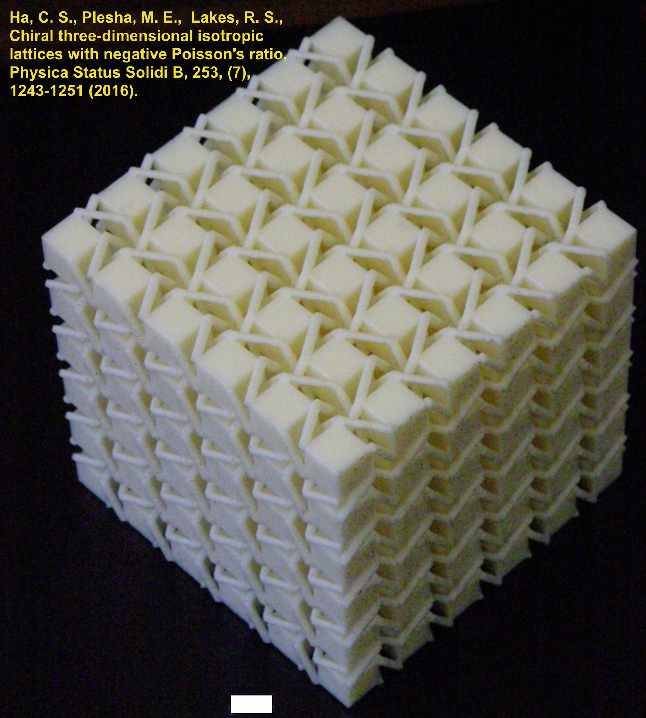Ha, C. S., Plesha, M. E., Lakes, R. S., "Chiral three-dimensional isotropic lattices with negative Poisson's ratio", Physica Status Solidi B, 253, (7), 1243-1251 (2016). DOI: 10.1002/pssb.201600055
Chiral three-dimensional isotropic cubic lattices with rigid cubical nodules and multiple deformable ribs are developed and analyzed via finite element analysis. The lattices exhibit geometry dependent Poisson's ratio that can be tuned to negative values. Poisson's ratio decreases from positive to negative values as the number of cells increases. Isotropy is obtained by adjustment of aspect ratio. The lattices exhibit significant size effects. Such a phenomenon cannot occur in a classical elastic continuum but it can occur in a Cosserat solid.
This is the first three-dimensional metamaterial that is chiral; we did not call it by such a name.
preprint pdf

Li, D. Dong, L., Lakes R. S., "A unit cell structure with tunable Poisson's ratio from positive to negative", Materials Letters, 164, 456-459 (2016).
A novel unit cell structure with re-entrant hollow skeleton is designed and its Poisson's ratio has been studied using the finite element method (FEM) as a function of the geometric variables and the parent material Poisson's ratio. The simulation results showed that the Poisson's ratio of the unit cell structure is tunable over the full range of Poisson's ratio (-1 to +0.5) by varying the geometric variables and parent material Poisson's ratio. Three types of samples with identical geometric variables were fabricated via 3D printing using three different materials, and their Poisson's ratios were measured and compared with the ones from FE simulation, and excellent agreement was found between the experimental results and FE simulation.
preprint pdf

Li, D. Ma, J., Dong, L., and Lakes, R. S., "A bi material structure with Poisson's ratio tunable from positive to negative via temperature control", Materials Letters, 181, 285-288 15 October (2016).
In this paper, a two-dimensional quadrilateral cellular structure made from bi-material strips was designed and its thermal deformation behaviors were studied via experimental, analytical and numerical approaches. It has been shown that the cell shape of the structure can be tuned from convex to concave (or vice versa) and hence the Poisson's ratio from positive to negative (or vice versa) with a change in temperature. At any specific temperature with a non-zero T, the absolute value of the structure's Poisson's ratio decreased rapidly at first with an increasing compression strain in the y direction and then more slowly as it approached a constant of approximately 1 when the strain in the y direction is greater than 0.1. A maximum absolute value of the Poisson's ratio of approximately 12 was found for strain in the y direction for a 10 deg C temperature change.
preprint pdf

Li, D. Dong, L., Yin, J., Dong, L. and Lakes, R. S., "Numerical analysis on mechanical behaviors of hierarchical cellular structures with negative Poisson's ratio", Smart Materials and Structures, 26 025014 (7pp) (2017)
A novel 2D cellular structure achieved by alternating rectangular surface indents on the vertical ribs of the unit cells was designed. And the Poisson's ratios of several structures were determined using the finite element method (FEM) as a function of geometric parameters. The simulation results showed that the Poisson's ratio of the 2D cellular structure was sensitive to the cell dimensional ratio, and the value can be tuned from positive to negative(the minimum can reach -1.05). Samples with identical geometric variables were fabricated via 3D printing, and their Poisson's ratios were measured and compared with the ones from simulation. Excellent agreement was found between the experimental results and the simulation. The stiffness of the model can be higher than honeycomb made of regular hexagons with appropriate geometric parameters.
preprint pdf

Li, D., Ma, J., Dong, L. Lakes, R. S., "Stiff square structure with a negative Poisson's ratio", Materials Letters, 188, 149-151 1 February (2017). http://www.sciencedirect.com/science/article/pii/S0167577X16317608 http://dx.doi.org/10.1016/j.matlet.2016.11.036
A novel 2D cellular structure achieved by alternating rectangular surface indents on the vertical ribs of the unit cells was designed. And the Poisson's ratios of several structures were determined using the finite element method (FEM) as a function of geometric parameters. The simulation results showed that the Poisson's ratio of the 2D cellular structure was sensitive to the cell dimensional ratio, and the value can be tuned from positive to negative (the minimum can reach -1.05). Samples with identical geometric variables were fabricated via 3D printing, and their Poisson's ratios were measured and compared with the ones from simulation. Excellent agreement was found between the experimental results and the simulation. The stiffness of the model can be higher than honeycomb made of regular hexagons with appropriate geometric parameters.

Lakes, R. S., "Negative-Poisson's-Ratio Materials: Auxetic Solids", Annual Review of Materials Research, 47: 63-81 July (2017).
Poisson's ratio had long been considered to be an intrinsic material property, confined within a narrow domain and governed solely by the geometry of interatomic bonds. Materials with designed heterogeneity allow control over the Poisson's ratio. Poisson's ratios of any value within the thermodynamically admissible domain may be attained, including negative Poisson's ratio (called auxetic). In this article, we discuss the role of Poisson's ratio in elasticity, two-dimensional and three dimensional materials, phase transformations, underlying causes in the microstructure and other negative physical properties.

Li, D., Ma, J., Dong, L. Lakes, R. S., "Three-dimensional stiff cellular structures with negative Poisson's ratio" Physica Status Solidi B. 254, (12) 1600785, 5 pages Dec. (2017).
A novel three-dimensional (3D) cellular structure with negative Poisson's ratio was designed by alternating cuboid surface indents on the vertical ribs of the unit cells. The Poisson's ratio and Young's modulus of structures with different geometric parameters were determined using the finite element method (FEM) as a function of these parameters. Samples with identical geometric variables were fabricated via 3D printing, and their through-thickness direction Poisson's ratio were measured and compared with simulation results. Results showed that the Poisson's ratio of the 3D cellular structures can be tuned from positive to negative. Good agreement was found between the experimental results and the simulation. This lattice structure is considerably stiffer than re-entrant negative Poisson's ratio foam with the same solid phase.
preprint pdf

Li, D., Yin, J., Dong, L. Lakes, R. S., "Strong re-entrant cellular structures with negative Poisson's ratio", J. Materials Science, 53, (5), 3493-3499 March (2018).
In this paper, two new 2D re-entrant topologies with negative Poisson's ratio are presented and their mechanical properties (Poisson's ratio and energy absorption capacity) are studied using finite element method as a function of geometric parameters. The first topology (model 1) was constructed by adding two sinusoidal-shaped ribs into the classical re-entrant topology, while the second topology (model 2) was made by introducing extra vertical ribs to reinforce the sinusoidal-shaped ribs. Simulation results show that model 1 and model 2 topologies can reach a minimum value in Poisson's ratio of - 1.12 and - 0.58 with an appropriate geometric aspect ratio, respectively. The energy absorption capacities of model 1, model 2 and classical re-entrant model were studied at various compression velocities. Enhanced energy absorption capacities were observed in the two new re-entrant topologies compared with the classical re- entrant topology. journal link.
preprint pdf

Andrade, C., Ha, C. S., Lakes, R. S., "Extreme Cosserat elastic cube structure with large magnitude of negative Poisson's ratio", Journal of mechanics of materials and structures (JoMMS), 13 (1) 93-101 (2018).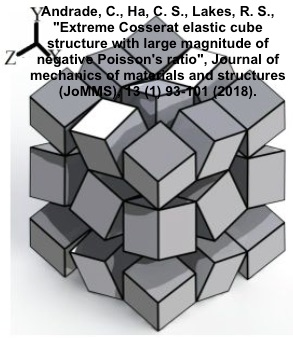journal link DOI: 10.2140/jomms.2018.13.93
preprint pdf
A structure consisting of pivoting cubes is presented. It has zero Young's modulus and zero bulk modulus. Poisson's ratio has large negative values in all directions; the structure exhibits anisotropy in Poisson's ratio. One may refer to anisotropic auxeticity. The structure is compliant in tension but rigid in torsion and bending. The Cosserat characteristic length tends to infinity.

Wenjiao, Z. , Neville, R., Dayi, Z., Scarpa, F., Lifeng, W., Lakes, R. S., The two-dimensional elasticity of a chiral hinge lattice metamaterial, International Journal of Solids and Structures, 141-142, 254-263, 1 June (2018). journal link
We present a lattice structure defined by patterns of slits that follow a rotational symmetry (chiral) configuration. The chiral pattern of the slits creates a series of hinges that produce deformation mechanisms for the lattice due to bending of the ribs, leading to a marginal negative Poisson's ratio. The engineering constants are modeled using theoretical and numerical Finite Element simulations. The results are benchmarked with experimental data obtained from uniaxial and off-axis tensile tests, with an overall excellent agreement. The chiral hinge lattice is almost one order of magnitude more compliant than other configurations with patterned slits and in contrast to other chiral micropolar media exhibits an in plane shear modulus that closely obeys the relation between Young's modulus and Poisson's ratio in homogeneous isotropic linear elastic materials.
preprint pdf

Rueger, Z., Ha, C. S. and Lakes, R. S., "Flexible cube tilt lattice with anisotropic Cosserat effects and negative Poisson's ratio" Physica Status Solidi B, 256, 1800512 (2019).
A 3D lattice structure consisting of pivoting cubes with flexible links at the corners is presented. It exhibits anisotropic negative Poisson's ratio -0.54 and -0.75. Size effects occur in torsion and bending; these effects are consistent with Cosserat elasticity but not with classical elasticity. Cosserat elastic solids exhibit sensitivity to strain gradients; size effects occur in torsion and bending; also reduction in stress concentrations. The Cosserat effects also reveal anisotropy.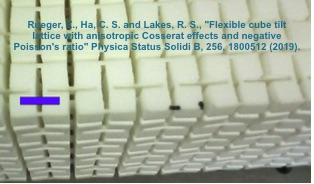preprint pdf

Q. Zhang, W. Lu, F. Scarpa, D. Barton, R. S. Lakes, Y. Zhu, Z. Lang, H. X. Peng, Large stiffness thermoformed open cell foams with auxeticity, Applied Materials Today, Volume 20, 100775, September (2020).

J. Li, C. S. Ha, R. S. Lakes, "Observation of squeeze twist coupling in a chiral three-dimensional isotropic lattice", Physica Status Solidi B Volume 257, Issue 10, 1900140, October (2020).
A chiral 3D lattice was designed, made by 3D printing, and studied experimentally. The lattice exhibited squeeze-twist coupling and a Poisson's ratio near zero. Squeeze-twist coupling does not occur in classical elasticity which makes no provision for chirality. By contrast, chiral effects are allowed in Cosserat elasticity. An experimental squeeze-twist coupling strain ratio on the order of unity and a Poisson's ratio near zero are in reasonable agreement with prior finite element analysis of a lattice with similar structure, for which negative Poisson's ratio is anticipated for a sufficient number of cells.

T. DeValk and R. S. Lakes, "Poisson's ratio and modulus of the gyroid lattice", Physica Status Solidi B 258 (12) 2100081 (2021). Gyroid surface lattices of different density exhibited Young's modulus consistent with stretch-dominated extremal behavior approaching the Hashin-Shtrikman upper bound. Gyroid lattices exhibited a Poisson's ratio of 0.34 independent of direction, independent of specimen diameter and independent of chirality. This behavior is in contrast with prior chiral lattices that exhibited pronounced size effects in Poisson's ratio, allowable for chiral elastic solids. doi link
preprint pdf

R. S. Lakes, "Extremal hinged lattices do not obey the theory of elasticity", Zeitschrift fur angewandte Mathematik und Physik (ZAMP) 73:27 (2022). Hinged lattices that attain extremal values of Poisson's ratio do not in general obey the theory of elasticity. Hinged structures that attain a Poisson's ratio of -1 are easy to stretch or deform volumetrically but they resist bending, in contrast to the predictions of elasticity theory. Their behavior corresponds to that of a Cosserat solid with divergent characteristic length. Hinged structures that attain a maximum Poisson's ratio are rigid with respect to hydrostatic tension, are easy to shear or to stretch but are unstable with respect to hydrostatic compression.

R. S. Lakes, B. Huey, and K. Goyal,"Extended Poisson's ratio range in chiral isotropic elastic materials", Physica Status Solidi B Volume 259, Issue 12, 2200336 (2022). Poisson's ratio in chiral Cosserat elastic solids is considered. Chirality allows the Poisson's ratio to exceed classical bounds, even if the material is directionally isotropic and all elastic moduli are within thermodynamic limits based on strain energy density. Poisson's ratio in chiral rods depends on the chiral elastic constants as well as on the shear and bulk moduli, assumed positive. Poisson's ratio can be greater than 0.5 or smaller than -1 for slender chiral specimens.
preprint pdf

T. DeValk, J. Hestetune, R. S. Lakes,"Nonclassical thermal twist of the chiral gyroid lattice", Physica Status Solidi B, Volume 259, Issue 12 2200338 (2022). The gyroid lattice was prepared in chiral and non-chiral forms. The chiral gyroid lattice was observed to exhibit temperature induced twist with direction of twist corresponding to the sense of chirality. This effect is a nonclassical effect that cannot occur in classical elasticity or classical thermo-elasticity but is allowable in Cosserat solids. Poisson's ratio of the gyroid is known to be about 0.3 with minimal dependence on size. In contrast to squeeze-twist coupling in which substantial size effects occur with slender specimens twisting much more than thicker ones, thermal-twist coupling exhibits opposite and less consistent size effects of much smaller magnitude.

R. S. Lakes, "Poisson's ratio beyond the classically allowable range in chiral isotropic elastic materials: effect of kappa and experiment", Physica Status Solidi B accepted (2023). doi link

Negative Poisson's ratio in deformed chicken wire. Positive Poisson's ratio, as one might expect, in normal chicken wire.

The effect of geometry on Poisson's ratio can also be explored using models based on LEGO blocks: MRSEC LEGO education book pdf.

The second international workshop on auxetic ( negative Poisson's ratio ) materials and related systems was held near Poznan, Poland in August 2005. Link 2005; group image courtesy Professor Peel. I am second from the right.
2007, Malta 4th International Workshop on Auxetics and Related Systems & 4th Annual AuxetNet Young Researchers Forum Link 2007
2009, 6th International Workshop on Auxetics and Related Systems, Bolton UK     Dry Link 2009 http://208.254.39.65/iomcomm/e_article001508037.cfm
2011, 8th International Workshop on Auxetics and Related Systems, Poznan, Poland Link 2011
2012, 4th International Conference and 9th International Workshop on Auxetics and Related Systems, Bolton, UK.     Dry Link 2012 http://www.bolton.ac.uk/Conferences/Auxetics2012/Home.aspx
2014, 5th International Conference and 10th International Workshop on Auxetics and Related Systems, Poznan, Poland Link 2014
2018, AUXETICS 2018, 9th International Conference and 14th International Workshop on Auxetics and Related Systems with "Negative" characteristics,    Dry Link 2018 https://blogs.shu.ac.uk/auxetics2018

imechanica blog node on auxetic materials

A special issue of Composites Science and Technology, 70, issue 7, 2010, was devoted to Chiral Smart Materials. The originating concept is the honeycomb of Prall and Lakes (1997), provided above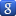Google bookmark

Top